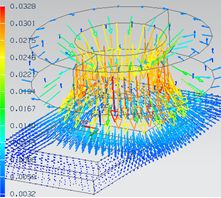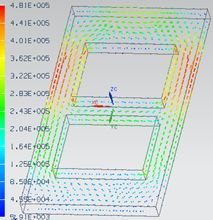## Magnetodynamics - Frequency-domainThe magneto-dynamic solution in frequency domain describes the distribution of magnetic fields and eddy currents due to time harmonic excitation loads.

We use a quasi stationary approximation. The assumptions are applicable in cases of dimensions << wavelength. Examples are motors, transformers and frequencies from 0 Hz to a few 100 kHz.

## Features

• 2D, 3D or axisymmetric Solution
• coupled with Thermal solution possible
• Outputs Plot:
• Magnetic Fluxdensity, Magnetic Fieldstrength, Current Density, Eddy Current Losses Density, Hysteresis-, Eddy-, Excess Loss Density - steinmetz, Magnetic Potential (a-Pot).
• Outputs Table:
• RotorBand Torque - stresstensor, Magnetic Potential on Conductors - Fluxlinkage, Voltage on Coils, Voltage on Circuits, Electrode Voltage, Electrode Current, Current on Circuits, Power on Circuits, Eddy Current Losses, Hysteresis-, Eddy-, Excess Losses, Ohm Resistance, Coil Inductivity, Phase Shift.

## Theory and Basics

### Formulations

The basis equations:
(1)        rot h = j
(2)        rot e = -δt b
(3)        div b = 0

Constitutive relations:
(4)        b = µ h
(5)        j = σ e

#### a-Formulation

The following a-formulation is used for 2D-Magnetodynamics.

Magnetic vectorpotential a:
(6)        b = rot a
(7)        e = -δt a

Magnetodynamic  weak  a-formulation:
(8)            ( µ-1 rot a, rot a’ )Ω
+ (-µ-1 bs, rot a’ ) Ω

+ (- ja’ ) ΩC
+ (σ δt aa’ ) Ωc

= 0, for all a’ element of Ω

#### a-v-Formulation

The following a-v-formulation is used for 3D-Magnetodynamics.

Magnetic vectorpotential a, electric scalar potential v:
(9)         b = rot a
(10)       e = -δt – grad v

Magnetodynamic  weak  a-v-formulation:
(11)           (µ-1 rot a, rot a’ )Ω
+ (σ δt aa’ ) Ωc
+ (σ grad v, a’ ) Ωc
+ (σ δt a, grad v’ ) Ωc
+ (σ grad v, v’ ) Ωc

= 0

### Basic Example: Team Problem 3: The Bath Plate

The problem 3 of the TEAM (Testing Electromagnetic Analysis Methods) is one of the examples for testing eddy current codes. A conductive plate with two holes is placed under a coil. The coil is driven by alternating current of 50 Hz and 1260 ampere turns. The goal is to analyze for the magnetic fluxdensity along a line that goes slightly over the plate.The Mesh is shown in the following picture.#### Results: Fluxdensity and  Induced Current

The magnetic fluxdensity as contourplotThe magnetics fluxdensity as vectorplotThe induced current density as contourpolotThe induced current density as vectorplotWe use cookies on our website. Some of them are essential for the operation of the site, while others help us to improve this site and the user experience (tracking cookies). You can decide for yourself whether you want to allow cookies or not. Please note that if you reject them, you may not be able to use all the functionalities of the site.